# Section 3.4. UWB Multiple-Access Techniques

### 3.4. UWB Multiple-Access Techniques

Ultra-wideband technology can deliver large amounts of data with low power spectral density and has been proven to be useful in short-range, high-data-rate applications. Such applications require that several transmitters coexist in the covered area. Therefore, proper multiple-access techniques are needed to perform the channelization for multiple users. In a typical multiple-access communications system, several users transmit information simultaneously and independently over a shared channel. The received signal is therefore the superposition of all users' signals, with different delay and attenuation factors caused by the wireless link, in addition to channel noise. A multiuser receiver extracts the desired user's signal from the received signal using proper demodulation techniques.

A major limiting factor on the performance of multiuser receivers is multiple-access interference, which is caused by the cross-correlation of unwanted users' signals on the desired signal due to their partial overlap. Figure 3-15 represents the block diagram of a typical multiple-access communications system.

##### Figure 3-15. A typical multiple-access communications system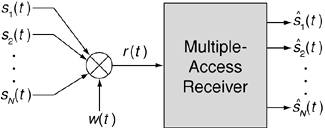In this figure, sk(t) and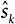(t) (where k equals 1 to N) represent the original and the estimated signals for the desired user, respectively; w(t) is the channel noise (usually considered as AWGN for theoretical analysis). Finally, r(t) is the received signal, which is a combination of the desired signal plus MAI and AWGN.

Equation 3-13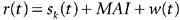The deteriorating effect of MAI is more severe in UWB systems due to their strict transmit power limitation, as set by the FCC. In this section we discuss how the UWB modulation techniques introduced in Section 3.3 can be used in multiple-access channels. As mentioned previously, OOK modulation is usually not considered for multiple-access channels due to its susceptibility to false messages by unwanted users. However, the PAM scheme can be modified for use in multiple-access channels by assigning different levels of amplitude for each user's data representation, as shown in Equation 3-14.

Equation 3-14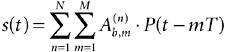where N represents the maximum number of users, and consequently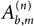is the specific power level for each user's data bits; M is the maximum number of transmitted bits; P(t) is the UWB pulse; bm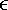[0,1] represents the T is the pulse repetition period.

A more complicated PAM technique is M-ary PAM, in which the information is modulated with ± M variations in signal amplitude. Note that the parameter M in M-ary PAM is different from the variable used for the number of bits in Equation 3-14. In other words, the amplitude of the transmitted signal is varied in discrete steps, with each level representing one or more bits for each user in a multiple-access channel.

The PPM scheme can also be modified to accommodate multiple users by assigning a unique modulation index, d, to each user. The general signal model for this version of the PPM-UWB signal in a multiple-access channel is given by

Equation 3-15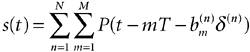where d(n) is the modulation index that provides a unique time shift to represent digital bits for each user, N represents the maximum number of users, M is the maximum number of transmitted bits, P(t) is the UWB pulse, bm[0,1] represents the T is the pulse repetition period.

Biphase modulation can also be used for multiple-access channels by assigning a unique code for each user (similar to the Code Division Multiple Access [CDMA] technique) and modulating pulses based on the assigned code. This way the data can be recovered by a template consisting of a set of pulses that match the specific user's code.

TR modulation is also a good candidate for UWB multiple-access communications. Assigning a unique delay for each user's TR doublet provides multiple-access capability for TR transceivers. The separation of each user in a TR-modulated UWB multiple-access system is controlled by the specific delay between pulses for each channel. The signal model for a TR-modulated signal in a multiple-access channel is

Equation 3-16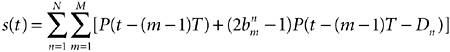where N represents the maximum number of users in the channel, Dn is the unique delay for user n,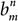is the mth data bit for the nth user, M is the maximum number of transmitted bits, P(t) is the UWB pulse, bm[0,1] represents the T is the pulse repetition period. Figure 3-16 shows an example of TR multiuser detection where the desired user is assumed to be User 2.

##### Figure 3-16. A two-user illustration of TR receivers. Data pulses are represented by solid lines, and reference pulses are represented by dotted lines for both users.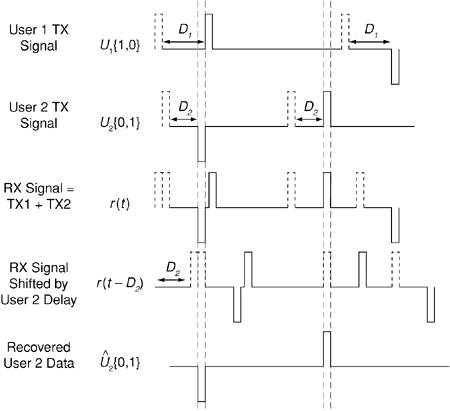To avoid catastrophic collisions in multiuser channels, a technique called time hopping can be used in conjunction with OOK, PAM, PPM, and biphase modulation. The next subsection introduces the time-hopping scheme used with PPM (TH-PPM); however, once the concept of time hopping is understood, all the previously mentioned modulation schemes can be considered instead of PPM. A concept similar to time hopping called delay hopping (DH-TR) is used for TR-modulated pulses in multiple-access channels. We discuss this scheme in Section 3.4.2.

#### 3.4.1. Pulse-Position Modulation with Time Hopping

In time hopping with PPM, a time frame, Tf, is divided into subunits called chips with duration Tc. In this method, each data bit is represented by a pulse, shifted based on d in a specific chip using a pseudorandom code in each time frame. Figure 3-17 shows an example of a two-user UWB TH-PPM technique. In this example, User 1 has a code word equal to C1 = [0 1 3] to represent its data bits (0 1 1) in dashed lines; User 2 uses a code word equal to C2 = [1 3 1] to transmit its data bits (1 0 1) in solid lines.

##### Figure 3-17. Pulse-position modulation with time hopping in a two-user channel.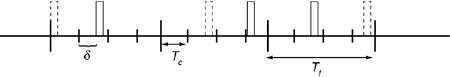The general signal model of a TH-PPM signal in a multiple-access channel is given by

Equation 3-17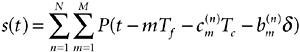where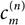is the code word or time-hopping sequence for each bit of the specific user's data and Tc is the chip duration. The parameter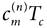adds an additional time shift to the PPM pulses to avoid catastrophic collisions, d is the modulation index that provides a unique time shift to represent digital bits, N represents the maximum number of users, M is the maximum number of transmitted bits, P(t) is the UWB pulse, bm[0,1]represents the T is the pulse repetition period.

#### 3.4.2. Delay-Hopped Transmitted-Reference Modulation

In this technique, a chip comprises a reference pulse with a fixed number of doublets; a set of delays known to both the transmitter and the receiver is used to represent each user in a multiple-access channel. This method is very similar to the CDMA technique for spread-spectrum communications. In DH-TR modulation, multiple pulses with multiple delays are used to represent the data. Hence, in order to distinguish different users in a multiple-access channel, the delay between the reference and the data pulses changes based on a fixed pattern known to the receiver. Figure 3-18 illustrates DH-TR modulation with a delay code of {D1, D2, D3, D4} and the delay-hopping pattern of {+1, +1, 1, 1} for a single-user system.

##### Figure 3-18. Delay-hopped transmitted-reference modulation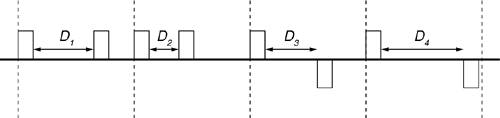The DH-TR receiver consists of a bank of pulse correlators that can detect a pulse pair with a specific delay. These correlators are followed by a delay-hopping code word correlator. The delay code word correlator samples the output of a series of pulse-pair correlators and adds them up based on prior knowledge of the code word at the receiver. If the received code word matches the expected code word, the data will be demodulated.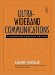Ultra-Wideband Communications: Fundamentals and Applications
ISBN: 0131463268
EAN: 2147483647
Year: 2005
Pages: 93

flylib.com © 2008-2017.
If you may any questions please contact us: flylib@qtcs.net